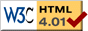# Classifying Differential Equations

previous next

When you study differential equations, it is kind of like botany. You learn to look at an equation and classify it into a certain group. The reason is that the techniques for solving differential equations are common to these various classification groups. And sometimes you can transform an equation of one type into an equivalent equation of another type, so that you can use easier solution techniques. Here then are some of the major classifications of differential equations:

On this page we assume that x and y are functions of time, t :

x = x(t)
y = y(t)

And the derivatives are with respect to t

 d x = x'(t) d t

## Partial vs. Ordinary

An ordinary differential equation (or ODE) has a discrete (finite) set of variables. For example in the simple pendulum, there are two variables: angle and angular velocity.

A partial differential equation (or PDE) has an infinite set of variables which correspond to all the positions on a line or a surface or a region of space. For example in the string simulation we have a continuous set of variables along the string corresponding to the displacement of the string at each position. In practice we approximate the infinite set of variables with a finite set of variables spread across the string (or surface or volume) at each position.

For an ODE, each variable has a distinct differential equation using "ordinary" derivatives. For a PDE, there is only one "partial" differential equation for each dimension.

## First Order, Second Order

The order of a differential equation is equal to the highest derivative in the equation. The single-quote indicates differention. So x' is a first derivative, while x'' is a second derivative.

x' = 1/x   is first-order

x'' = −x   is second-order

x'' + 2 x' + x = 0   is second-order

## Linear vs. Non-linear

Linear just means that the variable in an equation appears only with a power of one. So x is linear but x2 is non-linear. Also any function like cos(x) is non-linear.

In math and physics, linear generally means "simple" and non-linear means "complicated". The theory for solving linear equations is very well developed because linear equations are simple enough to be solveable. Non-linear equations can usually not be solved exactly and are the subject of much on-going research. Here is a brief description of how to recognize a linear equation.

Recall that the equation for a line is

y = m x + b

where m, b are constants ( m is the slope, and b is the y -intercept). In a differential equation, when the variables and their derivatives are only multiplied by constants, then the equation is linear. The variables and their derivatives must always appear as a simple first power. Here are some examples.

x'' + x = 0   is linear

x'' + 2x' + x = 0   is linear

x' + 1/x = 0   is non-linear because 1/x is not a first power

x' + x2 = 0   is non-linear because x2 is not a first power

x'' + sin(x) = 0   is non-linear because sin(x) is not a first power

x x' = 1   is non-linear because x' is not multiplied by a constant

Similar rules apply to multiple variable problems.

x' + y' = 0   is linear

x y' = 1   is non-linear because y' is not multiplied by a constant

Note, however, that an exception is made for the time variable t (the variable that we are differentiating by). We can have any crazy non-linear function of t appear in the equation, but still have an equation that is linear in x .

x'' + 2 x' + x = sin(t)   is linear in x

x' + t2x = 0   is linear in x

sin(t) x' + cos(t) x = exp(t)   is linear in x

See the Wikipedia article on linear differential equations for more details.

## Homogeneous vs. Non-homogeneous

This is another way of classifying differential equations. These fancy terms amount to the following: whether there is a term involving only time, t (shown on the right hand side in equations below).

x'' + 2_x' + x = 0   is homogeneous

x'' + 2_x' + x = sin(t)   is non-homogeneous

x' + t2x = 0   is homogeneous

x' + t2x = t + t2   is non-homogeneous

The non-homogeneous part of the equation is the term that involves only time. It usually corresponds to a forcing term in the physical model. For example, in a driven pendulum it would be the motor that is driving the pendulum.

This web page was first published June 2001.

previous next